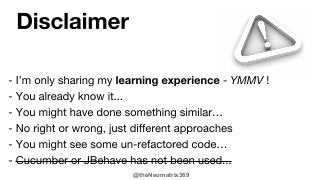Successfully reported this slideshow.×
1 of 56

Refactoring specifications

5

Share

In the past I have seen many a times, that only after we have delivered something to a client do we find out that we missed out on a feature or two. However tiny they might have been, they were mentioned in the spec. Nudged by this experience I have decided to take this seriously and develop a methodology for myself that will make my work closer to accurate than in the past.
In this session I will walk you through how I start with a simple piece of text (possibly obscure requirement), expand on it, and make it clearer till we can start writing code that will bring the specification to life. The example I show is of a mini-specification but the principles behind the technique shown, are universal and could be applied to other full-size specs as well.
I hope towards the end of the presentation, the code "hums" the same tune as the specification! And that you will be able to do something similar if not better, leaving with an additional tool in your tool-chest.
The presentation will be roughly 40% slides and 60% demo of code snippets. There won't be much live coding but a number of code snippets will be shown.

See all

See all

Refactoring specifications

1. 1. @theNeomatrix369
2. 2. @theNeomatrix369 ●●●●●
3. 3. @theNeomatrix369
4. 4. @theNeomatrix369 ●●●●●
5. 5. @theNeomatrix369
6. 6. @theNeomatrix369
7. 7. @theNeomatrix369
8. 8. @theNeomatrix369
9. 9. @theNeomatrix369 LSCC
10. 10. @theNeomatrix369
11. 11. @theNeomatrix369
12. 12. @theNeomatrix369
13. 13. @theNeomatrix369
14. 14. @theNeomatrix369
15. 15. @theNeomatrix369
16. 16. @theNeomatrix369
17. 17. @theNeomatrix369
18. 18. @theNeomatrix369
19. 19. @theNeomatrix369
20. 20. @theNeomatrix369
21. 21. @theNeomatrix369
22. 22. @theNeomatrix369
23. 23. @theNeomatrix369
24. 24. @theNeomatrix369
25. 25. @theNeomatrix369
26. 26. @theNeomatrix369
27. 27. @theNeomatrix369
28. 28. @theNeomatrix369
29. 29. @theNeomatrix369
30. 30. @theNeomatrix369
31. 31. @theNeomatrix369
32. 32. @theNeomatrix369
33. 33. @theNeomatrix369
34. 34. @theNeomatrix369
35. 35. @theNeomatrix369
36. 36. @theNeomatrix369
37. 37. @theNeomatrix369
38. 38. @theNeomatrix369
39. 39. @theNeomatrix369
40. 40. @theNeomatrix369
41. 41. @theNeomatrix369
42. 42. @theNeomatrix369
43. 43. @theNeomatrix369
44. 44. @theNeomatrix369 ●●●●●●●
45. 45. @theNeomatrix369 LSCC
46. 46. @theNeomatrix369
47. 47. @theNeomatrix369
48. 48. @theNeomatrix369 ● git clone git@github.com:neomatrix369/RefactoringSpecifications.git ●●●●●
49. 49. @theNeomatrix369
50. 50. @theNeomatrix369
51. 51. @theNeomatrix369
52. 52. @theNeomatrix369
53. 53. @theNeomatrix369
54. 54. @theNeomatrix369
55. 55. @theNeomatrix369